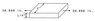# Help Me Find Max Tension/Shear Stress of Object

• misty777
Yes, at an uniaxial stress state, the maximal shear stress is \tau_{max} = \frac{\sigma}{2}.Great! Thanks a lot to everyone who helped me on this problem and for this wonderful site. I wish I had found it sooner!f

#### misty777

Hello, I am hoping that someone can help to point me in the right direction to solving the following problem.

I need to find the max tension stress and max shear stress of the object shown in the attachment and then compare those stresses with the ultimate tension stress of 64,000 psi and ultimate shear stress of 30,000 psi in order to determine the failure direction.

I am unable to detemine what forumla to use to begin with. I think I am right in understanding that to caluculate tension stress you would divide the load by the area. Is this the same as calculating maximum tension stress? Also if that is correct I am not given the length of the object in order to determine the area so I am unsure as to where to start. Any help is appreciated.

#### Attachments

•problem7.jpg
4.6 KB · Views: 364
You only need the area of the cross section.

Does this mean that I can use any variable for length of the cross section since I am not specifically given one?

Such as if I used 12in for the length I could determine the area of the object would be (12x2) + (12x2) + (.25x12) + (.25x12) = 54 sqin

Which would then be used in the formula to calculate the tension stress:
tension stress = 30,000/54
tension stress = 555.56psi

Am I on the right track here? How would knowing the tension stress then relate to finding the maximum tension stress?

With a uniform tension at the edge you get a u-----m tension stress at the clamped edge for an isotropic material. Shear stress is the same. You have no need for length here. Makes sense really.But, the directions are different. So the maximum is somewhere in between - I trust you'll find it with geometry's help.Just a note, if you have a perpendicular force at the edge then it's not just shear but aslo a moment - then things get a bit more complex because then the stress is not uniform any longer.

But, the directions are different. So the maximum is somewhere in between - I trust you'll find it with geometry's help.What maximum are you referring to? Under ideal assumptions, the normal force inside the bar is constant, i.e. N(x) = N, where x is the coordinate axe along the "unknown length". This makes the normal stress constant with a value of N/A, where A is the area of the cross section.

What maximum are you referring to? Under ideal assumptions, the normal force inside the bar is constant, i.e. N(x) = N, where x is the coordinate axe along the "unknown length". This makes the normal stress constant with a value of N/A, where A is the area of the cross section.
He said normal and shear.

I'm sorry I don't understand.

Force devided by area on which it acts gives stress. Uniform stress is when it's equal all over the relevant surface. According to Newton's first law, every action has a reaction so the force or stress acting on any crooss section of a beam is countered by an opposite force, which we assume is applied by a surface to which the beam is connected so it remains in place or the second law would apply and you'd have a free and accelerating beam section. Tension and shear both give you uniform stress, just that one acts along the beam and one perpendicular to the beam, respectively. From there you have 2 perpenidcular vectors with the length being the value of each force. Now find the maximum and it's direction.

Ok I'm going to take another shot at this one.

therefore stress = 30,000/.5
so the tension stress is 60,000 psi

Am I correct in that the maximum shear stress is equal to one-half of the maximum tensile stress which would make the shear stress 30,000 psi?

If I'm right this member will fail in shear because it has reached it's ultimate shear stress of 30,000 psi. Therefore the break would be at a 45 degree angle.

Yes, at an uniaxial stress state, the maximal shear stress is $\tau_{max} = \frac{\sigma}{2}$.

Great! Thanks a lot to everyone who helped me on this problem and for this wonderful site. I wish I had found it sooner!

Force devided by area on which it acts gives stress. Uniform stress is when it's equal all over the relevant surface. According to Newton's first law, every action has a reaction ...
That's Newton's 3rd law, but a good explanation anyways.

Tension and shear both give you uniform stress, just that one acts along the beam and one perpendicular to the beam, respectively.

Are you sure? I had 2 lectures on shear stresses in beams and if there's one thing my lecturer kept emphasizing it was that shear stresses are NOT UNIFORM across the face of a beam. Simply dividing the shear force by the area with give the average shear stress - potentially disastrous. In fact there was a collapse of a bridge in the 50s where a fresh graduate made this very mistake. Again, I'm just a first year and might have misunderstood what you're on about.

In this case it is uniform. If you were to twist the beam or have a moment act on it - then the shear is not uniform.

In this case it is uniform. If you were to twist the beam or have a moment act on it - then the shear is not uniform.

Ah right ofcourse. Cheers.